Skip to main content

# Qualitative analysis and sensitivity based optimal control of pine wilt disease

## Abstract

We design a deterministic model of pine wilt affliction to analyze the transmission dynamics. We obtain the reproduction number in unequivocal form, and global dynamics of the ailment is totally controlled by this number. With a specific end goal to survey the adequacy of malady control measures, we give the affectability investigation of basic reproduction number $$R_{0}$$ and the endemic levels of diseased classes regarding epidemiological parameters. From the aftereffects of the sensitivity analysis, we adjust the model to evaluate the effect of three control measures: exploitation of the tainted pines, preventive control to limit vector host contacts, and bug spray control to the vectors. Optimal analysis and numerical simulations of the model show that limited and appropriate utilization of control measures may extensively diminish the number of infected pines in a viable way.

## Introduction

Vector-borne illnesses are the maladies that outcome from disease transmitted by the nibble of infected arthropod species, for example, mosquitoes, fleas, ticks, and bugs. These biological agents that transmit contagious pathogen are called vectors. Malaria is the most regular case of vector-borne diseases. Many occurrences of vector-borne ailments are known for plants, for instance, coconut palm disease in palms and pine wilt illness in pine trees .

Pine wilt disease is a deadly ailment since it slays the infected tree within a few months. Bursaphelenchus xylophilus is the nematode that causes this disease. Monochamus alternatus, pine sawyer beetle, serves as a vector for this parasite, and it spreads the nematode to pine trees . It was first observed in 1905 in Japan. In United States, the pine wood nematode was first reported in 1934. Asian countries other than Japan began to report presence of pinewood nematode in the 1980s.

The first noticeable pine wilt disease symptom is reduction in the flow of oleoresin from bark wounds. Another indication of pine wilt disease is change of needle color from light grayish green to yellowish green, yellowish brown, and finally completely brown as tree succumbs to the disease .

Three transmission pathways of pine wilt disease are perceived. One occurs when adult beetles infested with nematode fly to healthy pine trees and begin maturation feeding and transmit nematode into the tree, and this transmission is pointed as a primary transmission. The secondary transmission occurs during egg lying activities of mature female on dead or dying, freshly cut pine tree. Horizontal transmission of nematode occurs during mating as mature male search for female beetle in bark wounds like oviposition wounds .

In this paper, we formulate a mathematical model based on ordinary differential equations. This model describes the infectious disease of pine trees through pine sawyer beetles. The motivation behind this paper is two-overlay. The first is to discuss the qualitative behavior of the proposed model. The second point is to accomplish awareness about the most attractive method for limiting the transmission of the disease using the sensitivity analysis. On the basis of sensitivity analysis, the model is modified by including three time-dependent controls: erosion of infected trees, tree-injection, and atmospheric pesticide spray.

## Model framework

We formulate a four-dimensional mathematical model composed of the susceptible host pine trees $$S_{h}$$ at time t that are at risk of being infected by the nematode. These trees radiate oleoresin that performs as a natural barrier to beetle oviposition, infected host pine tree $$I_{h}$$ at time t that have stopped exduating oleoresin, susceptible vector beetles $$S_{v}$$ at time t that do not have pinewood nematode, and the infected vector beetles $$I_{v}$$ at time t that carry pinewood nematode. The common transmission of nematodes among pine trees and bark beetles occur during maturation feeding of infected vectors. The pine sawyers have pinewood nematode when it emerges from infected pine trees. However, the beetles may likewise get tainted directly through copulating. Let $$N_{h}$$ denote total population of host pine trees, and let $$N_{v}$$ denote the total vector population consisting of adult beetles at any time t, respectively. Hence mathematically the populations are given by the equations $$N_{h}=S_{h}+I_{h}$$ and $$N_{v}= S_{v}+I_{v}$$.

Let $$\Pi_{h}$$ be the constant recruitment rate of pine trees at time t, and let $$\Pi_{v}$$ be the constant appearance rate of adults beetles at time t. We assume that the $$\delta_{1}$$ represent the transmission rate per contact during maturation feeding and $$\beta_{1}$$ accounts the average number of contacts per day with vector adult beetles during maturation feeding. The transmission rate of the nematode through infected vectors is denoted by $$\delta_{2}$$, and $$\beta_{2}$$ denotes the average number of contacts per day when adult beetles oviposit. The nematode carrying rate of adult beetles emerging from deceased trees is $$\beta_{3}$$. The incidence terms for the host population are $$\beta_{1}\delta_{1}S_{h}I_{v}$$ and $$\beta_{2}\delta_{2}\eta S_{h}I _{v}$$ during maturation feeding and oviposition, respectively. The incidence terms for vector population are $$\beta_{3}I_{h}S_{v}$$ and $$\beta S_{v}I_{v}$$, where β is the rate at which beetles pass on nematode during mating. The susceptible pine trees are exploiting at the rate $$\mu_{h}$$, the infected pine trees are isolating and felling at the rate σ, and $$\mu_{v}$$ is the death rate of vector population.

Under these assumptions, the mathematical model can be described as the following system of ordinary differential equations:

\begin{aligned}& \begin{aligned} &\frac{dS_{h}}{dt} =\Pi_{h}- \beta_{1} \delta_{1}S_{h}I_{v}- \beta_{2} \delta_{2}\eta S_{h}I_{v}- \mu_{h}S_{h}, \\ &\frac{dI_{h}}{dt} =\beta_{1}\delta_{1}S_{h}I_{v}+ \beta_{2}\delta _{2}\eta S_{h}I_{v}- \sigma I_{h}, \\ &\frac{dS_{v}}{dt} =\Pi_{v}-\beta_{3}S_{v}I_{h}- \beta S_{v}I_{v}-\mu _{v}S_{v}, \\ &\frac{dI_{v}}{dt} =\beta_{3}S_{v}I_{h}+\beta S_{v}I_{v}-\mu_{v}I _{v}. \end{aligned} \end{aligned}
(1)

Note that each described variable will remain nonnegative for nonnegative initial conditions because the model represents tree and beetle populations. The total vector population satisfies the following differential equation:

$$\frac{dN_{v}}{dt}=\Pi_{v}-\mu_{v}N_{v}.$$
(2)

This leads to $$N_{v}(t)\rightarrow \frac{\Pi_{v}}{\mu_{v}}$$ as $$t\rightarrow \infty$$. Thus, system (1) is reduced to the following three-dimensional system:

\begin{aligned}& \begin{aligned} &\frac{dS_{h}}{dt} =\Pi_{h}- \beta_{1} \delta_{1} S_{h}I_{v}- \beta_{2} \delta_{2}\eta S_{h}I_{v}- \mu_{h}S_{h}, \\ &\frac{dI_{h}}{dt} =\beta_{1}\delta_{1}S_{h}I_{v}+ \beta_{2}\delta_{2} \eta S_{h}I_{v}- \sigma I_{h}, \\ &\frac{dI_{v}}{dt} =(\beta_{3}I_{h}+\beta I_{v}) \biggl( \frac{\Pi_{v}}{\mu_{v}}-I_{v} \biggr)- \mu_{v}I_{v}. \end{aligned} \end{aligned}
(3)

The positively invariant region for system (3) is

$$\Delta = \biggl\{ (S_{h},I_{h},I_{v})\in R_{+}^{3} \Big\vert \frac{\Pi_{h}}{\sigma }\leq N_{h}\leq \frac{\Pi_{h}}{\mu_{h}}, 0 \leq I_{v}\leq \frac{\Pi_{v}}{\mu_{v}} \biggr\} ,$$

where $$R_{+}^{3}$$ represents the nonnegative part of $$R^{3}$$ including its lower-dimensional surfaces.

## Existence of equilibria

The disease dynamics is characterized by the basic reproduction, which is stated as ‘the average number of secondary infections produced by an infected individual in a completely susceptible population.’ The spread of the disease in a community is analyzed through the basic reproduction number. Its value for model (3) is given by

$$R_{0}= \frac{\beta \Pi_{v}}{\mu_{v}^{2}}+ \frac{\beta_{3}\Pi_{h}\Pi_{v} ( \beta_{1} \delta_{1} +\beta_{2} \delta_{2} \eta) }{\sigma \mu_{h}\mu_{v}^{2}}.$$
(4)

The disease-free equilibrium of system (3) is $$E_{0}=(\frac{ \Pi_{h}}{\mu_{h}},0,0)$$. Let $$E^{*}=(S_{h}^{*},I_{h}^{*},I_{v}^{*})$$ be the endemic equilibrium of model (3). The values of $$S_{h}^{*}$$ and $$I_{h}^{*}$$ are given by

\begin{aligned}& \begin{aligned} &S_{h}^{*}=\frac{\Pi_{h}-\sigma I_{h}^{*}}{\mu_{h}}, \\ &I_{h}^{*} =\frac{(\beta_{1}\delta_{1}+\beta_{2}\delta_{2}\eta)\Pi _{h}I_{v}^{*}}{\sigma (\mu_{h}+(\beta_{1}\delta_{1} +\beta_{2}\delta _{2}\eta)I_{v}^{*})}, \end{aligned} \end{aligned}
(5)

and $$I_{v}^{*}$$ is calculated by the quadratic equation

$$AI_{v}^{*2}+BI_{v}^{*}+C=0,$$
(6)

where

\begin{aligned}& \begin{aligned} &A =\beta \sigma (\beta_{1} \delta_{1}+ \beta_{2}\delta_{2}\eta), \\ &B =\beta_{3}\Pi_{h}(\beta_{1} \delta_{1}+\beta_{2}\delta_{2}\eta)+ \beta \sigma \mu_{h}+ (\beta_{1}\delta_{1}+\beta_{2} \delta_{2}\eta) \mu_{v}\sigma -\beta (\beta_{1} \delta_{1} +\beta_{2}\delta_{2}\eta) \frac{ \sigma \Pi_{v}}{\mu_{v}}, \\ &C =\mu_{v}\mu_{h}\sigma (1-R_{0}). \end{aligned} \end{aligned}
(7)

From (7) the following observations have been made:

• $$C<0$$ if and only if $$R_{0}>1$$.

• A is always positive.

• $$B>0$$ for $$R_{0}<1$$.

By the preceding it can be concluded that $$I_{v}^{*}$$ has no positive value for $$R_{0}<1$$ and unique positive value whenever $$R_{0}>1$$. We conclude the observations as follows.

### Theorem 3.1

An infection-free equilibrium $$E_{0}$$ of system (3) always exists, and a unique endemic equilibrium $$E^{*}= (S_{h}^{*} , I_{h} ^{*} , I_{v}^{*})$$ represented in (5) and (6) exists whenever $$R_{0}>1$$.

## Stability of equilibria

### Theorem 4.1

If $$R_{0}\leq 1$$, then the disease-free equilibrium $$E_{0}$$ of model (3) is globally asymptotically stable in Δ.

### Proof

Consider the following Lyapunov function:

\begin{aligned} V(t)=\alpha_{1}I_{h}+\alpha_{2}I_{v}, \quad \mbox{where } \alpha_{1}=\frac{\beta_{3}\Pi_{v}}{\mu_{v}}, \alpha_{2}= \sigma. \end{aligned}
(8)

The derivative of V along the solution of (3) is

\begin{aligned} V^{\prime } =&\alpha_{1}I_{h}^{\prime }+ \alpha_{2}I_{v}^{\prime } \\ =&\alpha_{1} \bigl[(\beta_{1}\delta_{1}+ \beta_{2}\delta_{2}\eta)S_{h}I _{v}- \sigma I_{h}\bigr]+\alpha_{2} \biggl[(\beta_{3}I_{h}+ \beta I_{v}) \biggl(\frac{\Pi _{v}}{\mu_{v}}-I_{v} \biggr)- \mu_{v}I_{v} \biggr] \\ \leq &\alpha_{1} \biggl[(\beta_{1}\delta_{1}+ \beta_{2}\delta_{2}\eta)\frac{ \Pi_{h}I_{v}}{\mu_{h}}-\sigma I_{h} \biggr] \\ &{}+\alpha_{2} \biggl[(\beta_{3}I_{h}+ \beta I_{v}) \biggl(\frac{\Pi_{v}}{\mu_{v}}-I_{v} \biggr)- \mu_{v}I_{v} \biggr] \\ =& \biggl[\alpha_{1}(\beta_{1}\delta_{1}+ \beta_{2}\delta_{2}\eta)\frac{\Pi _{h}}{\mu_{h}}- \alpha_{2}(\beta_{3}I_{h}+\beta I_{v})- \alpha_{2}\mu _{v} \biggr]I_{v} \\ &{}- \alpha_{1}\sigma I_{h}+\alpha_{2}( \beta_{3}I_{h}+\beta I _{v}) \frac{\Pi_{v}}{\mu_{v}} \\ =& \biggl[\alpha_{1 }(\beta_{1}\delta_{1}+ \beta_{2}\delta_{2}\eta)\frac{\Pi _{h}}{\mu_{h}}- \alpha_{2}\beta_{3}I_{h}-\alpha_{2}\beta I_{v}-\alpha _{2}\mu_{v}+\alpha_{2} \beta \frac{\Pi_{v}}{\mu_{v}} \biggr]I_{v} \\ &{}+\alpha_{2} \beta_{3}I_{h}\frac{\Pi_{v}}{\mu_{v}}-\alpha_{1}\sigma I_{h} \\ =&\alpha_{2}\mu_{v} \biggl[\frac{\alpha_{1}}{\alpha_{2}}( \beta_{1}\delta _{1}+\beta_{2}\delta_{2} \eta)\frac{\Pi_{h}}{\mu_{h}\mu_{v}}+\frac{ \beta \Pi_{v}}{\mu_{v^{2}}}-1 \biggr]I_{v} \\ &{}- \alpha_{2}\beta_{3}I_{h}I_{v}- \alpha_{2}\beta I_{v}^{2}+\alpha_{2} \beta_{3}I_{h}\frac{\Pi_{v}}{\mu _{v}}-\alpha_{1}\sigma I_{h} \\ =&\sigma \mu_{v}I_{v}(R_{0}-1)-\sigma \beta_{3}I_{h}I_{v}-\sigma \beta I_{v}^{2}. \end{aligned}

It can be seen that, for $$R_{0} \leq 1$$, we have $$V^{\prime }<0$$. Hence by Lyapunove’s first theorem $$E_{0}$$ is globally asymptotically stable in Δ. □

### Global stability of endemic equilibrium

When the threshold parameter $$R_{0} > 1$$, the uniform persistence of (3) can be proved by applying the technique given in , and the global stability of unique endemic equilibrium $$E^{\ast }$$ can be proved by using the technique of geometrical approach developed by Li and Muldowney . The geometric approach applied to host-vector models can be studied in [7, 8].

### Theorem 4.2

()

Suppose that $$H_{1}$$, $$H_{2}$$, and $$H_{3}$$ hold. The unique endemic equilibrium $$E^{\ast }$$ is globally stable in Δ if $$\bar{q} _{2}<0$$.

Clearly, $$\Delta =\{(S_{h},I_{h},I_{v})\in R_{+}^{3}\vert 0\leq N_{h} \leq \frac{\Pi_{h}}{\mu_{h}}, 0\leq I_{v}\leq \frac{\Pi_{v}}{\mu_{v}} \}$$ is a simply connected region, so $$H_{1}$$ holds. The boundedness of ξ and Lemma 5.1 given in  imply that system (3) has a compact absorbing set $$K \subset \Delta$$. Thus $$H_{2}$$ holds. $$H_{3}$$ holds in the view of Theorem 3.1. The appropriate vector norm $$\vert x\vert$$ in $$R^{3}$$ has been chosen together with the matrix-valued function $$P(x)=\operatorname{diag}(1,\frac{I_{h}}{I_{v}},\frac{I_{h}}{I_{v}})$$ of order $$3\times 3$$.

The function P is $$C^{1}$$ and nonsingular in the interior of Δ. The Jacobian matrix $$J=\frac{\partial f}{\partial x}$$, where f denotes the vector field, of system (3) is

$J= [ − μ h − ( β 1 δ 1 + β 2 δ 2 η ) I v 0 − ( β 1 δ 1 + β 2 δ 2 η ) S h ( β 1 δ 1 + β 2 δ 2 η ) I v − σ ( β 1 δ 1 + β 2 δ 2 η ) S h 0 β 3 ( Π v μ v − I v ) β Π v μ v − β 3 I h − 2 β I v − μ v ] .$
(9)

The second compound matrix of Jacobian is given by

$J [ 2 ] = [ b 11 ( β 1 δ 1 + β 2 δ 2 η ) S h ( β 1 δ 1 + β 2 δ 2 η ) S h ( Π v μ v − I v ) β 3 b 22 0 0 ( β 1 δ 1 + β 2 δ 2 η ) I v b 33 ] ,$

where,

\begin{aligned}& b_{11}=-\mu_{h}-\sigma -(\beta_{1} \delta_{1}+\beta_{2}\delta_{2} \eta)I_{v}, \\& b_{22}=-\mu_{h}-(\beta_{1}\delta_{1}+ \beta_{2}\delta_{2}\eta)I_{v}- \beta_{3}I_{h}+\frac{\beta \Pi_{v}}{\mu_{v}}-2\beta I_{v}- \mu_{v}, \\& b_{33}=-\beta_{3}I_{h}+\frac{\beta \Pi_{v}}{\mu_{v}}-2\beta I_{v}-\mu _{v}-\sigma . \end{aligned}

The block form of the matrix $$A=P_{f}P^{-1}+PJ^{}P^{-1}$$ is $$A= \bigl({\scriptsize\begin{matrix}{} A_{11} & A_{12} \cr A_{21} & A_{22} \end{matrix}} \bigr)$$ with

$A 11 = − σ − μ h − ( β 1 δ 1 + β 2 δ 2 η ) I v , A 12 = ( I v S h ( β 1 δ 1 + β 2 δ 2 η ) I h I v S h ( β 1 δ 1 + β 2 δ 2 η ) I h ) , A 21 = ( I h β 3 ( Π v μ v − I v I v 0 ) , A 22 = ( M 22 + I h ′ I h − I v ′ I v 0 ( β 1 δ 1 + β 2 δ 2 η ) I v M 33 + I h ′ I h − I v ′ I v ) , M 22 = − μ h − ( β 1 δ 1 + β 2 δ 2 η ) I v − β 3 I h + β Π v μ v − 2 β I v − μ v , M 33 = − β 3 I h + β Π v μ v − 2 β I v − μ v − σ .$

Let the norm in $$R^{3}$$ be defined as $$\vert (v_{1},v_{2},v_{3})\vert = \max ({\vert v_{1}\vert ,\vert v_{2}\vert +\vert v_{3}\vert })$$, where $$(v_{1},v_{2},v_{3})$$ represents the vector in $$R^{3}$$. The Lozinskiĭ measure regarding this norm is defined to be $$\mu (A)\leq \sup (g_{1},g_{2})$$, where

\begin{aligned}& g_{1}=\vert A_{12}\vert +\mu_{1}(A_{11}), \qquad g_{2}=\vert A_{21}\vert + \mu_{1}(A_{22}). \end{aligned}

System (3) can be written as

\begin{aligned}& \begin{aligned} &\frac{1}{I_{h}}\frac{dI_{h}}{dt} =(\beta_{1} \delta_{1}+\beta_{2} \delta_{2}\eta) \frac{S_{h}}{I_{h}}I_{v}-\sigma, \\ &\frac{1}{I_{v}}\frac{dI_{v}}{dt} = \biggl(\frac{\beta_{3}I_{h}+\beta I_{v}}{I _{v}} \biggr) \biggl( \frac{\Pi_{v}}{\mu_{v}}-I_{v} \biggr)-\mu_{v}. \end{aligned} \end{aligned}
(10)

The Lozinskiĭ measure of $$A_{11}$$ regarding any vector norm in $$R^{1}$$ will be $$A_{11}$$ because it is a scalar. Hence

\begin{aligned}& A_{11}=-\sigma -(\beta_{1}\delta_{1}+ \beta_{2}\delta_{2}\eta)I_{v}- \mu_{h}, \qquad \vert A_{12}\vert =\frac{I_{v}S_{h}(\beta_{1}\delta _{1}+\beta_{2}\delta_{2}\eta)}{I_{h}}, \end{aligned}

and $$g_{1}$$ will become

\begin{aligned} g_{1} =&-\sigma -\mu_{h}-(\beta_{1} \delta_{1}+\beta_{2}\delta_{2} \eta)I_{v}+ \frac{I_{v}S_{h} (\beta_{1}\delta_{1}+\beta_{2}\delta_{2} \eta)}{I_{h}} \\ =&\frac{I_{h}^{\prime }}{I_{h}}-(\mu_{h}+\beta_{1} \delta_{1}+\beta _{2}\delta_{2}\eta)I_{v}. \end{aligned}

Also, $$\vert A_{21}\vert =\beta_{3}\frac{I_{h}}{I_{v}}(\frac{\Pi_{v}}{ \mu_{v}}-I_{v})$$, $$\vert A_{12}\vert$$ is the operator norm of $$A_{12}$$ from $$R^{2}$$ to R, and $$\vert A_{21}\vert$$ is the operator norm of $$A_{21}$$ from R to $$R^{2}$$, and further $$R^{2}$$ is endowed with the $$l_{1}$$ norm. The Lozinskiĭ measure of $$\mu_{1}(A_{22})$$ of a matrix $$A_{22}$$ regarding $$l_{1}$$ norm in $$R^{2}$$ is

\begin{aligned} \mu ( A_{22} ) =&\sup \biggl\{ M_{22}+ \frac{I_{h}^{\prime }}{I_{h}}+( \beta_{1}\delta_{1}+\beta_{2}\delta _{2} \eta)I_{v}- \frac{I_{v}^{\prime }}{I_{v}},M_{33}+ \frac{I_{h}^{\prime }}{I_{h}}- \frac{I_{v}^{\prime }}{I_{v}} \biggr\} \\ =&\sup \biggl\{ -\mu_{h}-(\beta_{1}\delta_{1}+ \beta_{2}\delta_{2}\eta)I _{v}- \beta_{3}I_{h}+\frac{\beta \Pi_{v}}{\mu_{v}}-2\beta I_{v}-\mu _{v}+ \frac{I_{h}^{\prime }}{I_{h}} \\ &{}- \biggl(\frac{\beta_{3}I_{h}+\beta I_{v}}{I_{v}} \biggr) \biggl(\frac{\Pi_{v}}{\mu _{v}}-I_{v} \biggr)+\mu_{v}+(\beta_{1}\delta_{1}+ \beta_{2}\delta_{2} \eta)I _{v} , - \beta_{3}I_{h}+\frac{\beta \Pi_{v}}{\mu_{v}} \\ &{}-2\beta I_{v}-\mu_{v}-\sigma + \frac{I_{h}^{\prime }}{I_{h}}- \biggl(\frac{\beta_{3}I_{h}+\beta I_{v}}{I _{v}} \biggr) \biggl(\frac{\Pi_{v}}{\mu_{v}}-I_{v} \biggr)+ \mu_{v} \biggr\} \\ =& \frac{I_{h}^{\prime }}{I_{h}}- \biggl(\mu_{h}+\beta I_{v}+ \beta_{3}\frac{I _{h}\Pi_{v}}{I_{v}\mu_{v}} \biggr). \end{aligned}

Thus,

\begin{aligned} g_{2} =& \frac{I_{h}^{\prime }}{I_{h}}- \biggl(\mu_{h}+\beta I_{v}+\beta_{3}\frac{I _{h}\Pi_{v}}{I_{v}\mu_{v}} \biggr)+ \beta_{3} \frac{I_{h}}{I_{v}} \biggl(\frac{\Pi _{v}}{\mu_{v}}-I_{v} \biggr) \\ =& \frac{I_{h}^{\prime }}{I_{h}}-(\mu_{h}+\beta I_{v}+ \beta_{3} I_{h}). \end{aligned}

So

\begin{aligned} \mu (A) =&\sup \{g_{1},g_{2}\} \\ =&\sup \biggl\{ \frac{I_{h}^{\prime }}{I_{h}}-(\mu_{h}+\beta_{1} \delta_{1}+ \beta_{2}\delta_{2}\eta)I_{v}, \frac{I_{h}^{\prime }}{I_{h}}-(\mu_{h}+\beta I_{v}+ \beta_{3} I_{h}) \biggr\} \\ =& \frac{I_{h}^{\prime }}{I_{h}}-\lambda, \end{aligned}

where $$\lambda =\min \{(\mu_{h}+\beta_{1}\delta_{1}+\beta_{2}\delta _{2}\eta)I_{v},(\mu_{h}+\beta I_{v}+\beta_{3} I_{h}\}$$. Because (3) is uniformly persistent whenever $$R_{0}>1$$, for $$T>0$$, there exists $$t>T$$ such that $$I_{h}(t)\geq c$$ and $$I_{v}(t)\geq c$$. Also, $$\frac{1}{t}\log I_{h}(t)< \frac{\lambda }{2}$$ for all $$(S_{h0},I_{h0},I_{v0})\in K$$. Thus

$$\frac{1}{t} \int_{0}^{t}\mu (A)\,dt< \frac{\log I_{h}(t)}{t}-\lambda < - \frac{\lambda }{2}$$

for all $$(S_{h0},I_{h0},I_{v0})\in K$$, which further implies that $$\bar{q}_{2}<0$$. Since all the conditions of Theorem 4.2 are satisfied, the unique endemic equilibrium $$E^{\ast }$$ is globally asymptotically stable in Δ.

## Sensitivity analysis

We are interested in identifying important aspects for disease transmission and prevalence. In this manner, we can try to curtail significant economic losses caused by this disease. The reproductive number causes initial disease transmission, whereas disease prevalence depends upon the endemic equilibrium point. The class of infectious pines and vectors are the most important classes because pine forest destruction depends on these two classes. The sensitivity indices of the reproduction number and the endemic equilibrium will be calculated. This calculation is carried out with reference to parameters given in Table 1 for the model. The sensitivity indices analysis identifies the parameters that are more pivotal for disease transmission and prevalence.

### Definition

The normalized forward sensitivity index of a variable h that depends on the differentiability with respect to a parameter l is defined as $$\gamma_{l}^{h}=\frac{ \partial h}{\partial l}\times \frac{l}{h}$$. The sensitivity indices of $$R_{0}$$, $$I_{h}^{*}$$, and $$I_{v}^{*}$$ are given in Table 2.

By the analysis of sensitivity indices the most sensitive parameter is $$\mu_{v}$$. The reproduction number $$R_{0}$$ is inversely connected to $$\mu_{v}$$. Thus, it can be said that an increase (or decrease) in $$\mu_{v}$$ by 10%, $$R_{0}$$ decreases (or increases) by 20%. Similarly if we increase (or decrease) σ by 10%, then $$R_{0}$$ will also decrease (or increase) by 10%.

The endemic level of infected pine trees is inversely related to the mortality rate of bark beetles and exploitation rate of infected pine trees. We see that $$I_{h}^{*}$$ is decreased (increased) by almost four times with respect to the parameter $$\mu_{v}$$, and it is decreased (increased) almost 27% by increasing (decreasing) the exploitation rate by 10%.

The endemic level of infected vectors is again inversely related to the mortality rate of bark beetles and exploitation rate of infected pine trees. We observe that $$I_{v}^{*}$$ is decreased (increased) by almost five times with respect to the parameter $$\mu_{v}$$, and it is decreased (increased) almost 23% by increasing (decreasing) the exploitation rate by 10%.

The sensitivity indices of $$R_{0}$$, $$I_{h}^{\ast }$$, and $$I_{v}^{ \ast }$$ proposed that three controls, nematicide injected into the trunk of uninfected trees, cutting down infected trees burning and burying, and spray of insecticides, can be applied for vector control.

## Optimal control analysis

Now model (1) is modified to evaluate the effect of few control measures, namely nematicide injected into the trunk of uninfected trees, exploiting and burying infected pine trees, and spray of insecticides. In the pine population, the factor $$1-u_{1}$$ is involved to reduce the associated force of infection, and the exploitation rate of infected pine trees is increased at a rate $$u_{2}$$. The reproduction rate of the beetle population is reduced through the factor $$1-u_{3}$$.

\begin{aligned}& \begin{aligned} &\frac{dS_{h}}{dt} =\Pi_{h}-(1-u_{1}) ( \beta_{1}\delta_{1}+\beta_{2} \delta_{2} \eta) S_{h}I_{v}-\mu_{h}S_{h}, \\ &\frac{dI_{h}}{dt} =(1-u_{1}) ( \beta_{1} \delta_{1}+\beta_{2} \delta_{2}\eta) S_{h}I_{v}-\sigma I_{h}-r_{1}u_{2}I_{h}, \\ &\frac{dS_{v}}{dt} =\Pi_{v}(1-u_{3})-\beta_{3}S_{v}I_{h}- \beta S_{v}I _{v}- ( \mu_{v}+r_{0}u_{3} ) S_{v}, \\ &\frac{dI_{v}}{dt} =\beta_{3}S_{v}I_{h}+\beta S_{v}I_{v}- ( \mu _{v}+r_{0}u_{3} ) I_{v}. \end{aligned} \end{aligned}
(11)

The control function $$u_{1}$$ represents the use of nematicide injected into the trunk of uninfected trees. The control function $$u_{2}$$ represents the increase in exploitation rate of infected pine trees so that bark beetle could not oviposit on them. The level of adulticide used for vector control such as aerial spraying of pesticide is represented by the control function $$u_{3}$$. Thus the reproduction rate of the vector population is diminished by a factor of $$1 - u_{3}$$. Further, we assume that the exploitation rate of infected pine trees and the mortality rate of the vector population increase at rates proportional to $$r_{1}$$ and $$r_{0}$$, respectively.

For the disease control, it is necessary to examine the optimal level of efforts. For this purpose, we design the objective functional J. This objective functional helps us in minimizing the number of infected pines and also the expense of applying the controls $$u_{1}$$, $$u_{2}$$, $$u _{3}$$:

$$J= J(u_{1},u_{2},u_{3s}) = \int_{0}^{T} \biggl(A_{1}I_{h}+A_{2}N_{v}+ \frac{1}{2}B_{1}u_{1}^{2} + \frac{1}{2}B_{2}u_{2}^{2}+ \frac{1}{2}B_{3}u _{3}^{2} \biggr)\,dt,$$
(12)

where $$A_{1}$$ and $$A_{2}$$ are positive weights. We shall find an optimal solution $$u^{*}_{1}$$, $$u^{*}_{2}$$, $$u^{*}_{3}$$ satisfying

$$J \bigl(u_{1}^{\ast },u_{2}^{\ast },u_{3}^{\ast } \bigr)=\min \bigl\{ J(u_{1},u_{2},u _{3}):u_{1},u_{2},,u_{3} \in U \bigr\} ,$$
(13)

where $$U=\{(u_{1},u_{2},u_{3})\}$$ is the control set, and $$0\leq u _{i}\leq 1$$ ($$i=1,2,3$$) are measurable. Pontryagin’s maximum principle  is used to satisfy the necessary conditions of the optimal solution. For the application of this principle, we define the Hamiltonian H as

\begin{aligned} H(X,U,\lambda) =&A_{1}I_{h}+A_{2}N_{v}+ \frac{B_{1}}{2}u_{1}^{2}+\frac{B _{2}}{2}u_{2}^{2}+ \frac{B_{3}}{2}u_{3}^{2} \\ &{}+\lambda_{1} \bigl[ \Pi_{h}-(1-u _{1}) ( \beta_{1}\delta_{1}+ \beta_{2}\delta_{2}\eta) S_{h}I _{v}- \mu_{h}S_{h} \bigr] \\ &{}+\lambda_{2} \bigl[ (1-u_{1}) ( \beta_{1} \delta_{1}+\beta_{2} \delta_{2}\eta) S_{h}I_{v}- ( \sigma +r_{1}u_{2} ) I _{h} \bigr] \\ &{}+\lambda_{3} \bigl[ \Pi_{v}(1-u_{3})- \beta_{3}S_{v}I_{h}-\beta S_{v}I _{v}- ( \mu_{v}-r_{0}u_{3} ) S_{v} \bigr] \\ &{}+\lambda_{4} \bigl[ \beta_{3}S_{v}I_{h}+ \beta S_{v}I_{v}- ( \mu _{v}+r_{0}u_{3} ) I_{v} \bigr] . \end{aligned}

Here $$\lambda_{i}$$, $$i=1,2,3,4$$, are the adjoint variables. To prove the existence of the optimal control using the result given by Fleming and Rishel , we state and prove the following theorem.

### Theorem 6.1

There exists an optimal control $$(u_{1}^{\ast },u_{2}^{\ast },u_{3} ^{\ast })$$ that minimizes J over U subject to the control system (11). Further, for system (11), there exist adjoint variables $$\lambda_{i}$$, $$i=1,2,3,4$$, satisfying

\begin{aligned}& \begin{aligned}&\frac{d\lambda_{1}}{dt} = ( \lambda_{1}-\lambda_{2} ) (1-u _{1}) (\beta_{1}\delta_{1}I_{v}+ \beta_{2}\delta_{2}\eta I_{v})+\lambda _{1}\mu_{h}, \\ &\frac{d\lambda_{2}}{dt} =-A_{1}+\lambda_{2}(\sigma +r_{1}u_{2})+ ( \lambda_{3}-\lambda_{4} ) (1-u_{1})\beta_{3}S_{v}, \\ &\frac{d\lambda_{3}}{dt} =-A_{2}+(\lambda_{3}- \lambda_{4}) (\beta_{3}I _{h}+\beta I_{v})+\lambda_{3} ( \mu_{v}+r_{0}u_{3} ), \\ &\frac{d\lambda_{4}}{dt} =-A_{2}+ ( \lambda_{1}- \lambda_{2} ) (\beta_{1}\delta_{1}S_{h}+ \beta_{2}\delta_{2}\eta S_{h})+ ( \lambda_{3}-\lambda_{4} ) \beta S_{v}- \lambda_{4}(\mu_{v}+r_{0}u _{3}), \end{aligned} \end{aligned}
(14)

together with slanting conditions $$\lambda_{i}(T)=0$$ ($$i=1,2,3,4$$). The optimal controls are given by

\begin{aligned}& \begin{aligned} &u_{1}^{\ast } =\max \biggl\{ \min \biggl\{ 1, \frac{ ( \lambda_{2}-\lambda_{1} ) ( \beta_{1}\delta_{1}+\beta_{2} \delta_{2}\eta) S_{h}I_{v}}{B_{1}} \biggr\} ,0 \biggr\} , \\ &u_{2}^{\ast } = \max \biggl\{ \min \biggl\{ 1,\frac{\lambda_{2}r_{1}I_{h}}{B_{2}} \biggr\} ,0 \biggr\} , \\ &u_{3}^{\ast } =\max \biggl\{ \min \biggl\{ 1,\frac{\lambda_{3} ( \Pi_{v}-r_{0}S _{v} ) +\lambda_{4}r_{0}I_{v}}{B_{3}} \biggr\} ,0 \biggr\} . \end{aligned} \end{aligned}
(15)

### Proof

The function $$A_{1}I_{h}+A_{2}N_{v}+\frac{B_{1}}{2}u_{1}^{2}+\frac{B _{2}}{2}u_{2}^{2}+\frac{B_{3}}{2}u_{3}^{2}$$ is a convex function of $$u_{1}$$, $$u_{2}$$, $$u_{3}$$. Since the state solutions are bounded, the state system satisfies the Lipschitz property corresponding to the state variables. The existence of optimal control follows from . The equations representing the rate of change of the adjoint variables are formed by the differentiation of the Hamiltonian function with respect to state variables evaluated at the optimal control. The optimal solution given by (15) can be obtained by solving the equations

$$\frac{\partial H}{\partial u_{1}}=\frac{\partial H}{\partial u_{2}}=\frac{ \partial H}{\partial u_{3}}=0$$
(16)

on the internal of the control set using the property of the control space U. □

## Numerical simulations

In this section, we numerically solve the model. We observe that our numerical results are in good agreement with our theoretical results. Figure 1 shows that the population approaches the disease-free equilibrium when the reproductive number is less than 1, whereas Figure 2 shows that the population approaches endemic equilibrium as the reproduction number exceeds unity.Figure 1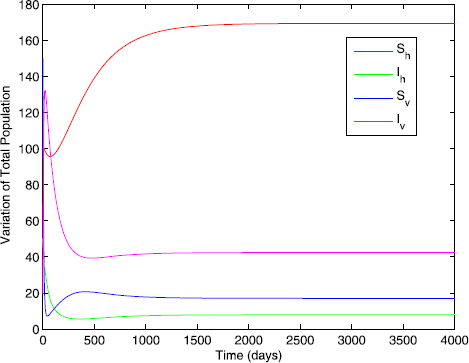Figure 2

Now, we investigate numerical results for the efficacy of the optimal control planning for the disease spread in a community. We have chosen the set of weight factors $$A_{1}=1$$, $$A_{2}=5$$, $$B_{1}=3$$, $$B_{2}=7$$, $$B_{3}=9$$ and initial state variables $$S_{h}(0)=100$$, $$I_{h}(0)=50$$, $$S_{v}(0)=150$$, $$I_{v}(0)=20$$ and $$r_{0}=0.55$$, $$r_{1}=0.1$$. The other parameter values are given in Table 1. We investigate numerically the effect of the following optimal control strategies.

### Application of preventive measures $$(u_{1}\neq 0)$$ and exploiting and disposing off infected pines $$(u_{2}\neq 0)$$

The objective functional J is optimized by applying the control $$u_{1}$$ on the susceptible pine trees through nematicide injections and exploitation and disposing off infected pine trees $$u_{2}$$. Figure 3 shows that there is a significant difference in the number of infected pine trees by applying control and without control, but the number of infected vectors is not significantly reduced. The results shown in Figure 4 suggest that it is not a very effective strategy to control the number of infected vectors. The control profile explored in Figure 5 states that the control $$u_{1}$$ remains at the upper bound till 35 days, whereas the control $$u_{2}$$ rises to the upper bound after 60 days.Figure 3Figure 4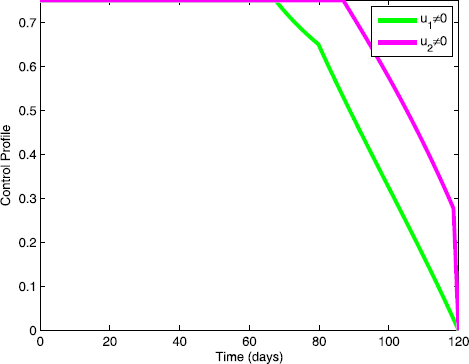Figure 5

### Application of preventive measures $$(u_{1}\neq 0)$$ and spray of insecticides $$(u_{3}\neq 0)$$

In this policy, we use the preventive control $$u_{1}$$ and spray of insecticides $$u_{3}$$ to optimize the objective functional J. In Figures 6 and 7, we observe a significant difference in the number of infected pines and infected vectors, respectively. By the analysis of control profile shown in Figure 8 we see that the control $$u_{1}$$ rises to its upper bound in 20 days, and after these days it gradually drops down to zero, whereas the control $$u_{3}$$ can be activated after 20 days.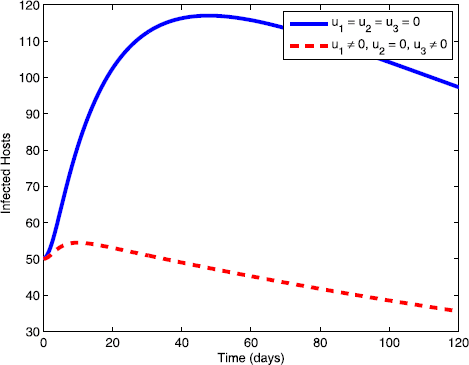Figure 6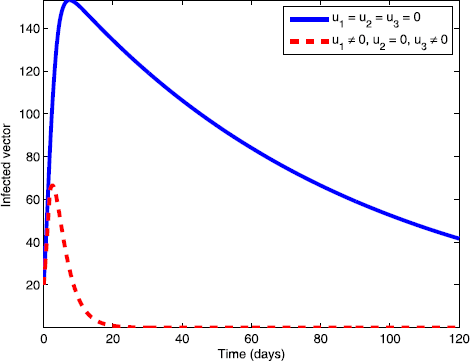Figure 7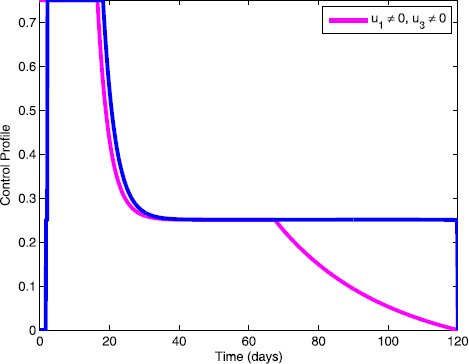Figure 8

### Use of exploitation of infected pines $$(u_{2}\neq 0)$$ and spray of insecticides $$(u_{3}\neq 0)$$

Here the objective function J is optimized by applying the controls $$u_{2}$$ and $$u_{3}$$. Figures 9 and 10 show that decrease in the number of infected pine trees and infected vectors occurs by applying these controls. The control profile of these controls is shown in Figure 11.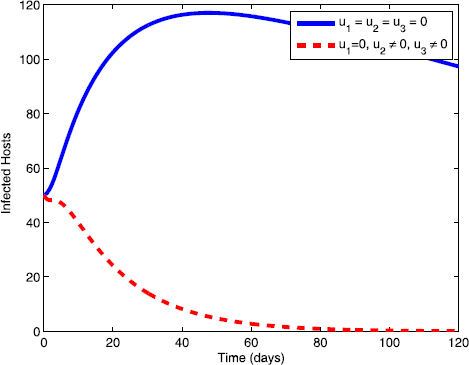Figure 9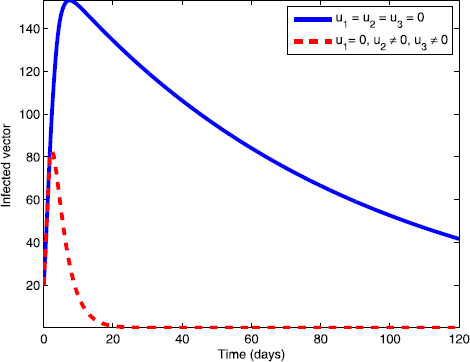Figure 10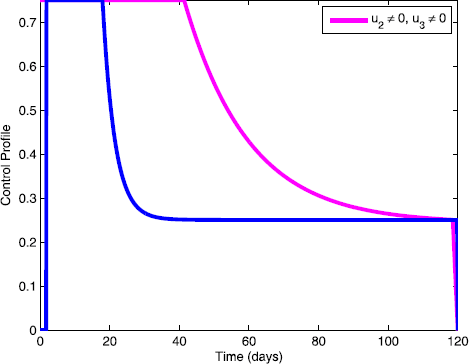Figure 11

### Use of preventive measures $$(u_{1}\neq 0)$$, exploitation of infected pines $$(u_{2}\neq 0)$$ and spray of insecticides $$(u_{3}\neq 0)$$

With this strategy, all the controls are used to optimize the objective function J. From Figures 12 and 13 we can see that this control strategy results in a significant decrease in the number of infected pines and infected vectors. The control profile for this control is shown in Figure 14.Figure 12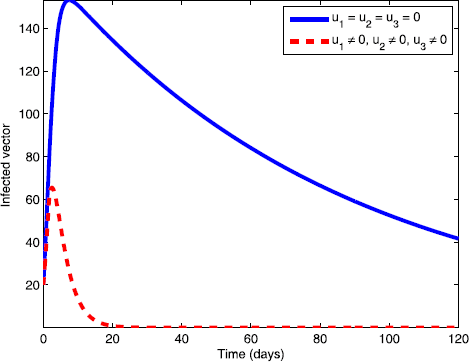Figure 13Figure 14

### Effects of weight constants

A sensitivity analysis is carried out by studying the adequacy of our simulations in relation to the weight constants and comparing the results with different weight constants on the controls $$u_{1}$$, $$u_{2}$$, and $$u_{3}$$. From Figures 15, 16, and 17 we see that as the value of weight constants increases, control functions decrease.Figure 15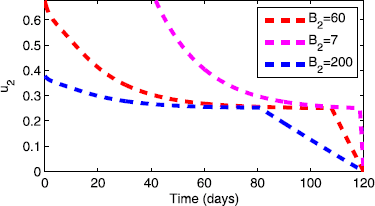Figure 16Figure 17

## References

1. 1.

Ozair, M, Lashari, AA, Jung, IH, Okosun, KO: Stability analysis and optimal control of a vector-borne disease with nonlinear incidence. Discrete Dyn. Nat. Soc. 2012, Article ID 595487 (2012).

2. 2.

Yoshimura, A, Kawasaki, K, Takasu, F, Togashi, K, Futai, K, Shigesada, N: Modeling the spread of pine wilt disease caused by nematodes with pine sawyers as vector. Ecology 80(5), 1691-1702 (1999)

3. 3.

Shi, X, Song, G: Analysis of the mathematical model for the spread of pine wilt disease. J. Appl. Math. 2013, Article ID 184054 (2013)

4. 4.

Edwards, OR, Linit, MJ: Transmission of Bursaphelenchus xylophilus through oviposition wounds of Monochamus carolinensis (Coleoptera: Cerambycidae). J. Nematol. 24, 133-139 (1992)

5. 5.

Ozair, M, Shi, X, Hussain, T: Control measures of pine wilt disease. Comput. Appl. Math. (2014). https://doi.org/10.1007/s40314-014-0203-2

6. 6.

Li, MY, Muldowney, JS: A geometric approach to global stability problem. SIAM J. Math. Anal. 27, 1070-1083 (1996)

7. 7.

Buonomo, B, Vargas-De-Leon, C: Stability and bifurcation analysis of a vector-bias model of malaria transmission. Math. Biosci. 242(1), 59-67 (2013)

8. 8.

Tumwiine, J, Mugisha, JYT, Luboobi, LS: A host vector model for malaria with infective immigrants. J. Math. Anal. Appl. 361(1), 139-149 (2010)

9. 9.

Lee, KS: Stability analysis and optimal control strategy for prevention of pine wilt disease. Abstr. Appl. Anal. 2014, Article ID 182680 (2014)

10. 10.

Togashi, K: Population density of Monochamus alternatus adults (Coleoptera: Cerambycidae) and incidence of pine wilt disease caused by Bursaphelenchus xylophilus (Nematoda:Aphelenchoididae). Res. Popul. Ecol. 30(2), 177-192 (1988)

11. 11.

Monserud, RA, Sterba, H: Modeling individual tree mortality for Austrian forest species. For. Ecol. Manag. 113(2-3), 109-123 (1999)

12. 12.

Kim, DS, Lee, SM, Huh, HS, Park, NC, Park, CG: Escape of pine wood Nematode, Bursaphelenchus xylophilus, through feeding and oviposition behavior of Monochamus alternatus and M.saltuarius (Coleoptera: Cerambycidae) adults. Korean J. Appl. Entomol. 48(4), 527-533 (2009)

13. 13.

Kobayashi, F, Yamane, A, Ikeda, T: The Japanese pine sawyer beetle as the vector of pine wilt disease. Annu. Rev. Entomol. 29, 115-135 (1984)

14. 14.

Kim, DS, Lee, SM, Kim, CS, Lee, DW, Park, CG: Movement of Monochamus altermatus hope (Coleoptera: Cerambycidae) adults among young black pine trees in a screen cage. Korean J. Appl. Entomol. 50(1), 1-6 (2011)

15. 15.

Pontryagin, LS, Boltyanskii, VG, Gamkrelidze, RV, Mishchenko, EF: The Mathematical Theory of Optimal Processes, vol. 4. Gordon & Breach, New York (1986)

16. 16.

Fleming, WH, Rishel, RW: Deterministic and Stochastic Optimal Control. Springer, New York (1975)

Download references

## Author information

Authors

### Contributions

All authors carried out the proofs of the main results and approved the final manuscript.

### Corresponding author

Correspondence to Muhammad Ozair.

## Ethics declarations

### Competing interests

The authors declare that they have no competing interests.

## Additional information

### Publisher’s Note

Springer Nature remains neutral with regard to jurisdictional claims in published maps and institutional affiliations.

## Rights and permissions

Open Access This article is distributed under the terms of the Creative Commons Attribution 4.0 International License (http://creativecommons.org/licenses/by/4.0/), which permits unrestricted use, distribution, and reproduction in any medium, provided you give appropriate credit to the original author(s) and the source, provide a link to the Creative Commons license, and indicate if changes were made.

Reprints and Permissions